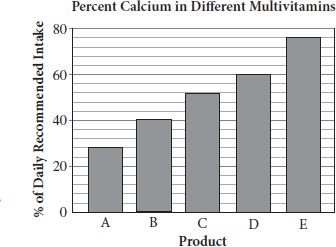# SAT Math Multiple Choice Question 842: Answer and Explanation

### Test Information

Question: 842

2.The bar graph above shows the percent of the daily recommended intake of calcium suppliedby five different multivitamins, A, B, C, D, and E, per pill. Products A and D aresold in bottles of 75 pills and cost \$12 and \$18, respectively. Products B, C, andE are sold in bottles of 50 pills and cost \$9.50, \$10.25, and \$15.50, respectively.Which of the five products supplies the greatest percentage of the daily-recommendedintake of calcium per dollar?

• A. A
• B. B
• C. C
• D. E

Explanation:

C

Difficulty: Hard

Category: Problem Solving and Data Analysis / Rates, Ratios, Proportions, and Percentages

Strategic Advice: Whenever you're comparing multiple rates (percent calcium per pill and percent calcium per dollar), especially when reading data from a graph, consider organizing the information in a table.

Getting to the Answer: The following table organizes the data provided by the graph and additional data needed to answer the question. Because there is more than one calculation to make per product, don't round any values until you reach the final column. (Note: Product D is not one of the answer choices, so save yourself a bit of time by skipping D in the calculations.)

ProductCost per bottleNumber of pillsCost per pill (not rounded)Percent of calcium supplied per pill (from the graph)Percent of calcium supplied per dollar (rounded to the nearest percent)
A\$12.007512 ÷ 75 = 0.1628%28 ÷ 0.16 = 175
B\$9.50509.5 ÷ 50 = 0.1940%40 ÷ 0.19 = 211
C\$10.255010.25 ÷ 50 = 0.20552%52 ÷ 0.205 = 254
E\$15.55015.5 ÷ 50 = 0.3176%76 ÷ 0.31 = 245

According to the calculations in the table, product C provides the greatest percentage of the daily-recommended intake of calcium per dollar, making (C) correct.